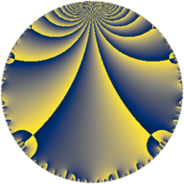# Properties

 Label 95.4.aLevel $95$ Weight $4$ Character orbit 95.a Rep. character $\chi_{95}(1,\cdot)$ Character field $\Q$ Dimension $18$ Newform subspaces $7$ Sturm bound $40$ Trace bound $3$

# Related objects

## Defining parameters

 Level: $$N$$ $$=$$ $$95 = 5 \cdot 19$$ Weight: $$k$$ $$=$$ $$4$$ Character orbit: $$[\chi]$$ $$=$$ 95.a (trivial) Character field: $$\Q$$ Newform subspaces: $$7$$ Sturm bound: $$40$$ Trace bound: $$3$$ Distinguishing $$T_p$$: $$2$$, $$3$$

## Dimensions

The following table gives the dimensions of various subspaces of $$M_{4}(\Gamma_0(95))$$.

Total New Old
Modular forms 32 18 14
Cusp forms 28 18 10
Eisenstein series 4 0 4

The following table gives the dimensions of the cuspidal new subspaces with specified eigenvalues for the Atkin-Lehner operators and the Fricke involution.

$$5$$$$19$$FrickeDim.
$$+$$$$+$$$$+$$$$6$$
$$+$$$$-$$$$-$$$$2$$
$$-$$$$+$$$$-$$$$3$$
$$-$$$$-$$$$+$$$$7$$
Plus space$$+$$$$13$$
Minus space$$-$$$$5$$

## Trace form

 $$18 q + 4 q^{2} + 52 q^{4} + 10 q^{5} + 68 q^{6} + 28 q^{7} - 48 q^{8} + 166 q^{9} + O(q^{10})$$ $$18 q + 4 q^{2} + 52 q^{4} + 10 q^{5} + 68 q^{6} + 28 q^{7} - 48 q^{8} + 166 q^{9} + 8 q^{11} + 64 q^{12} + 16 q^{13} - 24 q^{14} - 40 q^{15} + 484 q^{16} + 104 q^{17} - 108 q^{18} + 80 q^{20} + 240 q^{21} + 208 q^{22} + 12 q^{23} + 620 q^{24} + 450 q^{25} - 868 q^{26} + 288 q^{27} - 124 q^{28} + 220 q^{29} - 120 q^{30} + 344 q^{31} - 1720 q^{32} - 496 q^{33} - 592 q^{34} + 320 q^{35} - 1200 q^{36} + 112 q^{37} + 228 q^{38} - 116 q^{39} - 1084 q^{41} - 2796 q^{42} + 248 q^{43} - 792 q^{44} - 390 q^{45} - 512 q^{46} + 2024 q^{47} - 940 q^{48} + 1854 q^{49} + 100 q^{50} + 216 q^{51} + 436 q^{52} - 2432 q^{53} - 204 q^{54} + 320 q^{55} - 104 q^{56} + 228 q^{57} - 1132 q^{58} - 928 q^{59} + 340 q^{60} + 68 q^{61} + 136 q^{62} + 1392 q^{63} + 1332 q^{64} - 480 q^{65} + 2168 q^{66} + 1544 q^{67} + 1564 q^{68} + 1232 q^{69} - 1600 q^{70} - 1080 q^{71} - 908 q^{72} + 1040 q^{73} - 584 q^{74} + 768 q^{77} - 2792 q^{78} - 544 q^{79} - 360 q^{80} + 2810 q^{81} + 3744 q^{82} + 512 q^{83} + 1312 q^{84} + 500 q^{85} + 3448 q^{86} + 876 q^{87} + 3196 q^{88} + 3300 q^{89} + 1952 q^{91} + 4604 q^{92} + 4560 q^{93} - 3176 q^{94} + 760 q^{95} - 2980 q^{96} + 2512 q^{97} - 380 q^{98} + 664 q^{99} + O(q^{100})$$

## Decomposition of $$S_{4}^{\mathrm{new}}(\Gamma_0(95))$$ into newform subspaces

Label Dim $A$ Field CM Traces A-L signs $q$-expansion
$a_{2}$ $a_{3}$ $a_{5}$ $a_{7}$ 5 19
95.4.a.a $1$ $5.605$ $$\Q$$ None $$0$$ $$4$$ $$-5$$ $$-22$$ $+$ $-$ $$q+4q^{3}-8q^{4}-5q^{5}-22q^{7}-11q^{9}+\cdots$$
95.4.a.b $1$ $5.605$ $$\Q$$ None $$3$$ $$-5$$ $$-5$$ $$-1$$ $+$ $-$ $$q+3q^{2}-5q^{3}+q^{4}-5q^{5}-15q^{6}+\cdots$$
95.4.a.c $1$ $5.605$ $$\Q$$ None $$3$$ $$7$$ $$5$$ $$11$$ $-$ $-$ $$q+3q^{2}+7q^{3}+q^{4}+5q^{5}+21q^{6}+\cdots$$
95.4.a.d $1$ $5.605$ $$\Q$$ None $$5$$ $$4$$ $$5$$ $$-32$$ $-$ $-$ $$q+5q^{2}+4q^{3}+17q^{4}+5q^{5}+20q^{6}+\cdots$$
95.4.a.e $3$ $5.605$ 3.3.1304.1 None $$-3$$ $$-11$$ $$15$$ $$-5$$ $-$ $+$ $$q+(-1-\beta _{1})q^{2}+(-4-\beta _{1}-\beta _{2})q^{3}+\cdots$$
95.4.a.f $5$ $5.605$ $$\mathbb{Q}[x]/(x^{5} - \cdots)$$ None $$-3$$ $$-4$$ $$25$$ $$72$$ $-$ $-$ $$q+(-1+\beta _{1})q^{2}+(-\beta _{1}-\beta _{3})q^{3}+(4+\cdots)q^{4}+\cdots$$
95.4.a.g $6$ $5.605$ $$\mathbb{Q}[x]/(x^{6} - \cdots)$$ None $$-1$$ $$5$$ $$-30$$ $$5$$ $+$ $+$ $$q-\beta _{1}q^{2}+(1-\beta _{1}-\beta _{5})q^{3}+(4+\beta _{2}+\cdots)q^{4}+\cdots$$

## Decomposition of $$S_{4}^{\mathrm{old}}(\Gamma_0(95))$$ into lower level spaces

$$S_{4}^{\mathrm{old}}(\Gamma_0(95)) \cong$$ $$S_{4}^{\mathrm{new}}(\Gamma_0(5))$$$$^{\oplus 2}$$$$\oplus$$$$S_{4}^{\mathrm{new}}(\Gamma_0(19))$$$$^{\oplus 2}$$